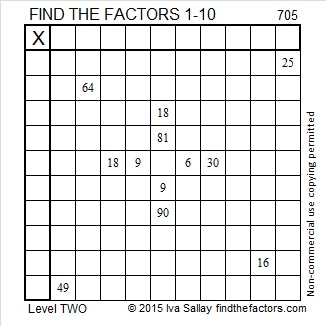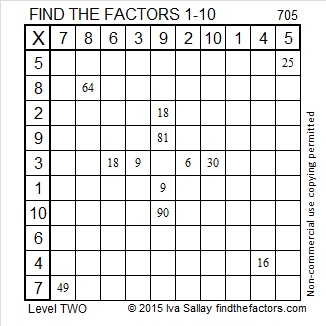# 705 and Level 2

• 705 is a composite number.
• Prime factorization: 705 = 3 x 5 x 47
• The exponents in the prime factorization are 1, 1, and 1. Adding one to each and multiplying we get (1 + 1)(1 + 1)(1 + 1) = 2 x 2 x 2 = 8. Therefore 705 has exactly 8 factors.
• Factors of 705: 1, 3, 5, 15, 47, 141, 235, 705
• Factor pairs: 705 = 1 x 705, 3 x 235, 5 x 141, or 15 x 47
• 705 has no square factors that allow its square root to be simplified. √705 ≈ 26.551836.Here is today’s puzzle:Print the puzzles or type the solution on this excel file: 10 Factors 2015-12-07

————————————————————————————

What else can I tell you about the number 705?

Because 5 is one of its factors, 705 is the hypotenuse of the Pythagorean triple 423-564-705. What is the greatest common factor of those three numbers?

705 is the sum of consecutive numbers several different ways:

• 352 + 353 = 705; (2 consecutive numbers)
• 234 + 235 + 236 = 705; (3 consecutive numbers)
• 139 + 140 + 141 + 142 + 143 = 705; (5 consecutive numbers)
• 40 + 41 + 42 + 43 + 44 + 45 + 46 + 47 + 48 + 49 + 50 + 51 + 52 + 53 + 54 = 705; (15 consecutive numbers)

705 is palindrome 1A1 in base 22; note that A is equivalent to 10 base 10, 22² = 484, and 1(484) + 10(22) + 1(1) = 705.

————————————————————————————This site uses Akismet to reduce spam. Learn how your comment data is processed.